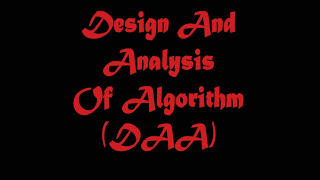# Principles of Analyzing algorithms and Problems | Algorithms Properties | Random Access Machine Model## Principles of Analyzing algorithms and Problems

An algorithm is a finite set of computational instructions, each instruction can be executed in finite time, to perform computation or problem solving by giving some value, or set of values as input to produce some value, or set of values as output. Algorithms are not dependent on a particular machine, programming language or compilers i.e. algorithms run in same manner everywhere. So the algorithm is a mathematical object where the algorithms are assumed to be run under machine with unlimited capacity.

### Examples of problems

• You are given two numbers; how do you find the Greatest Common Divisor
• Given an array of numbers, how do you sort them?

We need algorithms to understand the basic concepts of the Computer Science, programming. Where the computations are done and to understand the input output relation of the problem, we must be able to understand the steps involved in getting output(s) from the given input(s).

You need designing concepts of the algorithms because if you only study the algorithms then you are bound to those algorithms and selection among the available algorithms. However, if you have knowledge about design then you can attempt to improve the performance using different design principles.

The analysis of the algorithms gives a good insight of the algorithms under study. Analysis of algorithms tries to answer few questions like; is the algorithm correct? i.e. the Algorithm generates the required result or not?, does the algorithm terminate for all the inputs under problem domain? The other issues of analysis are efficiency, optimality, etc. So, knowing the different aspects of different algorithms on the similar problem domain we can choose the better algorithm for our need. This can be done by knowing the resources needed for the algorithm for its execution. Two most important resources are the time and the space. Both of the resources are measures in terms of complexity for time instead of absolute time we consider growth

### Algorithms Properties

Input(s)/output(s):
There must be some inputs from the standard set of inputs and an algorithm’s execution must produce outputs(s).

Definiteness:
Each step must be clear and unambiguous.

Finiteness:
Algorithms must terminate after finite time or steps.

Correctness:
Correct set of output values must be produced from each set of inputs.

Effectiveness:
Each step must be carried out in finite time. Here we deal with correctness and finiteness.

### Random Access Machine Model

This RAM model is the base model for our study of design and analysis of algorithms to have design and analysis in machine independent scenario. In this model each basic operations (+, -) takes 1 step, loops and subroutines are not basic operations. Each memory reference is 1 step. We measure run time of algorithm by counting the steps.